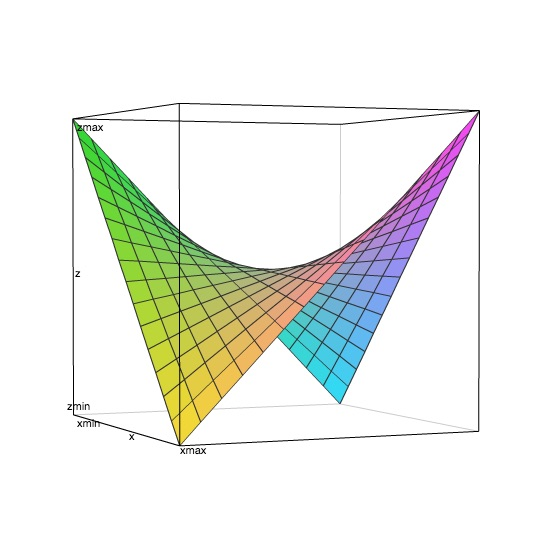# Pearl No.3 - Saddleback Search# Happy Easter

Our easter egg happens to be Pearl 3.

A function $f$ can have the following properties:

1. $f$ takes two arguments: $x$ and $y$
2. Both $x$ and $y$ are natural numbers, i.e., non-negative integers
3. $f$ also returns natural numbers
4. $f$ is strictly increasing in each argument. This means if $x$ increases or descreases, the according result of $f$ will also increase or descrease. The same applies on $y$.

Now we are given such a function $f$ and a natural number z, and we want to find out all pairs of $x$ and $y$ that makes $f (x, y) = z$.

In OCaml world, this problem requires us to write a function let find_all f z = ... which returns a list of (x, y) that satisfy f x y = z, assuming the supplied f meets the requirements above.

# Basic Math Analysis

This problem seems not that difficult. A trivial brute-force solution comes out immediately. We can simply try every possible value for $x$ and $y$ on $f$, and accumulate all $(x, y)$ satisfying $f (x, y) = z$ to the list. There is just one silly question:

Can we try all infinite $x$ and $y$?

Of course we cannot. We should try to set a range for both $x$ and $y$, otherwise, our solution would run forever. Then how? From some simple math analysis, we can conclude something about $f$, even if we won't know what $f$ will be exactly.

Here are what we know so far:

1. $x >= 0$
2. $y >= 0$
3. $z >= 0$
4. $x, y, z$ are all integers, which means the unit of increment or decrement is $1$
5. $f$ goes up or down whenever $x$ or $y$ goes up or down.
6. $f (x, y) = z$

From first 4 points, if we pick a value $X$ for $x$, we know we can descrease $x$ from $X$ at most $X$ times. It is also true for $y$ and $z$.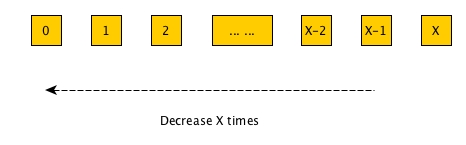Then let's assume $X$ and $Y$ makes $f$ equal to $Z$ ($X, Y, Z$ are assumed to be values), i.e.,

$$f (X, Y) = Z$$

Let's now fix $Y$ and try descreasing $x$ to $X-1$. From point 5, we know

$$f (X-1, Y) = Z_{X-1} < f (X, Y) = Z$$

We can continue to decrease $x$ until $0$:

$$f (0, Y) = Z_0 < f (1, Y) = Z_1 < ... < f (X-2, Y) = Z_{X-2} < f (X-1, Y) = Z_{X-1} < f (X, Y) = Z$$

Together with point 3 ($z >= 0$), we can simplify the above inequalities like:

$$0 <= Z_0 < Z_1 < ... < Z_{X-2} < Z_{X-1} < Z$$

We can see that through this descreasing of $x$, the number of results ($Z_0, Z_1, ..., Z_{X-1}$) is $X$. How many possible values between $0$ (inclusive) and $Z$ (exclusive)? Of course the answer is $Z$, right? So we can get $$0 <= X <= Z$$ and similarly, $$0 <= Y <= Z$$.

Thus, for any given $x, y, z$, their relationship is

$$0 <= x, y <= Z$$

We obtained the range and now our brute-force solution will work.

# Brute-force solution

It is simple enough.

let find_all_bruteforce f z =
let rec aux acc x y =
if y > z then aux acc (x+1) 0
else if x > z then acc
else
let r = f x y in
if r = z then aux ((x, y)::acc) x (y+1)
else aux acc x (y+1)
in
aux [] 0 0


The time complexity is $O(log(z^2))$. To be exact, we need $(z + 1)^2$ calculation of $f$. It will be a bit slow and we should seek for a better solution

# A Matrix

The fact that $f$ is an increasing function on $x$ and $y$ has been used to retrieve the range of $x$ and $y$. We actually can extract more from it.

If we fix the somewhat value of $y$, then increase $x$ from $0$, then the result of $f$ will increase and naturally be sorted. If we fix $x$, and increase $y$ from $0$, the same will happen that $f$ will increase and be sorted.

We also know $0 <= x, y <= Z$; thus, we can create a matrix that has $z + 1$ rows and $z + 1$ columns. And each cell will be the result of $f (x, y)$, where $x$ is the row number and $y$ is the column number.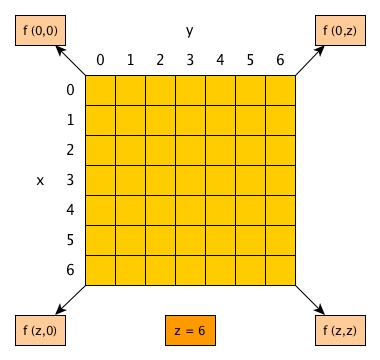The best thing from this matrix is that all rows are sorted and so are all columns. The reason is that $f$ is a strictly increasing function on $x$ and $y$.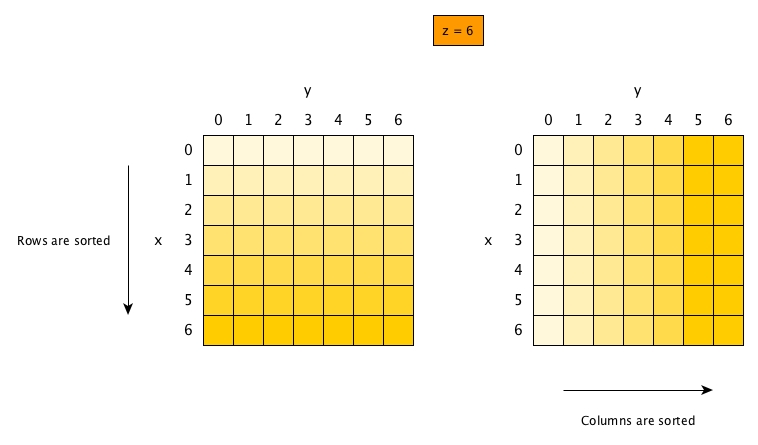This matrix converts the original problem to the one that now we have a board of unrevealed cards and we need to seek for an efficient strategy to find all cards that we want.

The trivial solution in the previous section has a simplest strategy: simply reveal all cards one by one and collect all that are satisfying.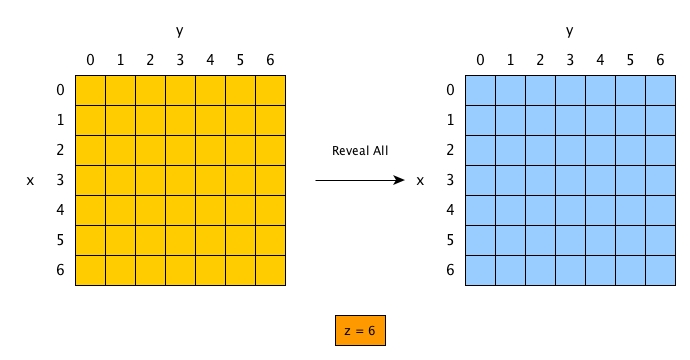# Zig-zag

Let's take an example simple function $f (x, y) = x * y$ and assume $z = 6$.

If we employ the trivial solution, then of course we will find all cards we want.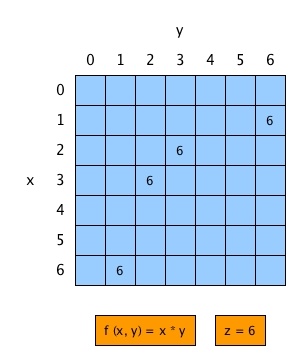However, we can see that we need to reveal $36$ cards for $4$ targets.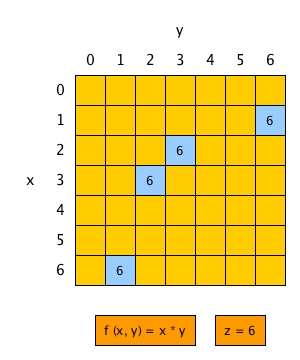What a waste! But how can we improve it?

Maybe just start from somewhere, reveal one card, depend on its value and then decide which next card to reveal?

Let's just try starting from the most natural place - the top-left corn, where $x = 0, y = 0$.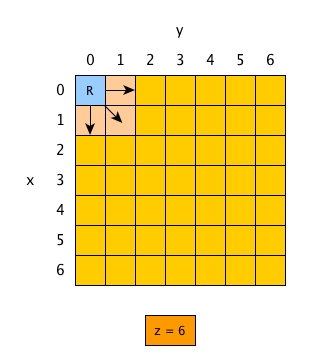1. We get $R$.
2. If $R > z$, our problem is solved. This is because the 3 possible next cards must be bigger than $R$ as the bigger $x$ or $y$ are the larger the results are. So we do not need to move any more.
3. What if $R < z$? then we have to try to reveal all 3 cards, which makes not much sense since we may still anyway reveal all cards.

We need a better way.

## Target sum of two sorted lists

Before we continue to think, let's try another simpler problem first.

Given a value k and two sorted lists of integers (all being distinct), find all pairs of integers (i, j) where i + j == k.

For example, [1; 2; 8; 12; 20] and [3; 6; 9; 14; 19] and k = 21 is given, we should return [(2, 19); (12, 9)].

Of course, we can just scan all possible pairs to see. But it is not efficient and we waste the execellent hint: sorted.

Since sorted list is the fundamental condition for merge, how about we try to do something similar? Let's put two sorted lists in parallel and for the first two elements $1$ and $3$, we know $1 + 3 = 4 < 21$.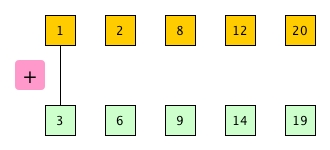It is too small at this moment, what should we do? We know we should move rightwards to increase, but do we move along list 1 or list 2 or both?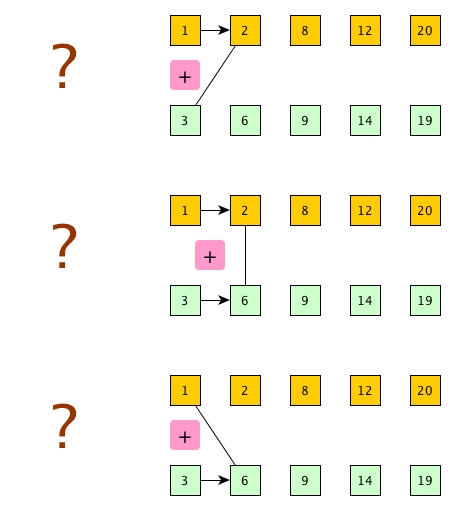We don't know actually, because each possible movement may give us chances to find good pairs. If we just take all possible movements, then it makes no sense as in the end as it will just try every possible pair. Hence, we just need to find a way to restrict our choices of movements.

How about we put one list in its natural order and put the other in its reversed order?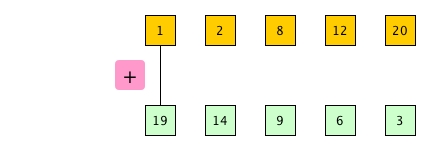Now, $1 + 19 = 20 < 21$. It is again too small. What shall we do? Can we move along list 2? We cannot, because the next element there is definitely smaller and if we move along, we will get even smaller sum. So moving along list 1 is our only option.

If i + j = k, then good, we collect (i, j). How about the next move? We cannot just move along any single list because the future sum will forever be either bigger than k or smaller. Thus, we move along both because only through increasing i and decreasing j may give us changes to find the target sum again.

What if i + j > k? It is easy to see that the only option for us is the next element in list 2.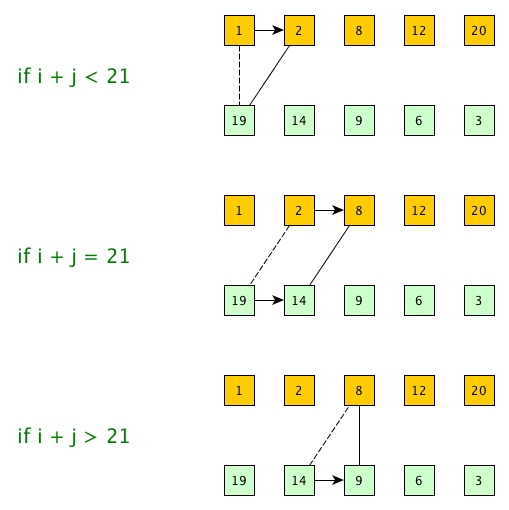## One ascending and the other descending, always

Let's come back to our sorted matrix problem. We do not have simple two sorted lists any more, but it is not difficult to see that we should start from bottom-left corner (rows are descending and columns are ascending). And depending on what we get from $R = f (x, y)$, we just change direction (either up, right-up or right), and towards the top-right corner:

1. We move right along $y$ until we reach the ceiling (the smallest number that is bigger than $z$), and then change to up
2. We move up along $x$ until we reach the floor (the bigger number that is smaller than $z$), and then change to right
3. Whenever we reach $R = f (x, y) = z$, we change to right-up.

We can use an example to explain why we want the ceiling (similarly explaining why the floor).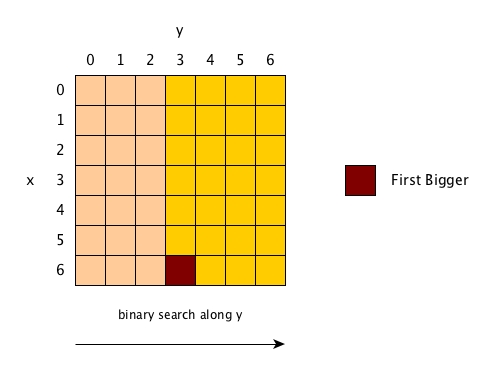When we reach the ceiling, we know all cells on its left can be dicarded because those are definitely smaller than $z$.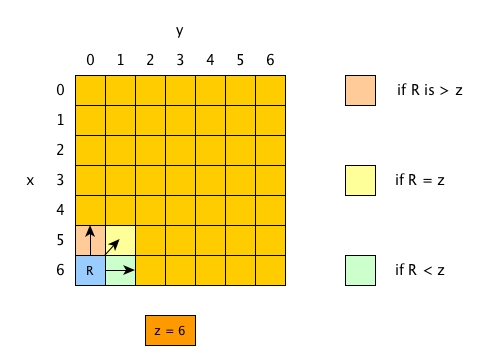In this way, the card revealing process for $f (x, y) = x * y$ looks like this: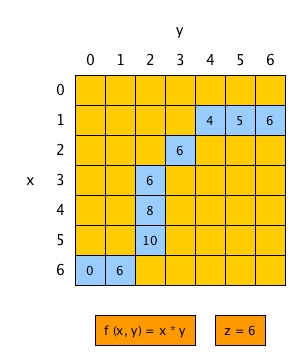## Code

let find_all_zigzag f z =
let rec aux acc x y =
if x < 0 || y > z then acc
else
let r = f x y in
if r < z then aux acc x (y+1)
else if r> z then aux acc (x-1) y
else aux (r::acc) (x-1) (y+1)
in
aux [] z 0


The time complexity is $O(Z)$ and in the worst case, we need to calculate $2 * Z + 1$ times of $f$.

This solution is a linear one and there is still room for improvement.

## A same problem with slightly different appearance

There is a quite popular interview question which is very similar to the pearl 3.

We have a matrix of numbers. All rows are sorted and so are all columns. Find the coordinates of a given number.

Although it seems all numbers are already computed and put in the matrix, both this problem and pearl 3 are actually identical.

# Zig-zag + Binary Search

Zig-zag solution is actually scan along $x$ axis and $y$ axis by turns. The scan on each turn is linear. But wait, each row and column are sorted, right? Does it ring a bell?

When a sequence of elements are sorted, and if we have a target to find, then we of course can try binary search.

Simply say, in order to improve the zig-zag solution, we just replace the linear scan part with binary search.

## Targets of binary search

For each round of binary search, we cannot just search for the given value of $z$ only. Instead,

1. Our target is the ceiling when we binary search horizontally, i.e., along $y$, from left to right.
2. Our target is the floor when we binary search vertically, i.e., along $x$, from down to up.
3. During our search, if we find a target card, we can simply stop.

## Eventually stops at ceiling or floor or equal

The next question is when a round of binary search stops?

When we reach an card equal to $z$, of course we can stop immediately.

If we never reach an ideal card, then anyway the search will stop because there will eventually be no room on either left side or right side to continue. And that stop point will definitely be the ceiling or the floor of $z$.

Again, because we need the ceiling for horizon and floor for vertical, we need to adjust it after we finish the binary search.

## Code

type direction = H | V

let rec binary_search z g p q =
if p + 1 >= q then p
else
let m = (p + q) / 2 in
let r = g m in
if r = z then m
else if r > z then binary_search z g p (m-1)
else binary_search z g (m+1) q

(* adjust ceiling or floor *)
let next_xy z f x y direction =
let r = f x y in
match direction with
| _ when r = z -> x, y
| H when r > z -> x-1, y
| H -> x-1, y+1
| V when r < z -> x, y+1
| V -> x-1, y+1

let find_all_zigzag_bs f z =
let rec aux acc (x, y) =
if x < 0 || y > z then acc
else
let r = f x y in
if r = z then aux (f x y::acc) (x-1, y+1)
else if r < z then
let k = binary_search z (fun m -> f x m) y z in
aux acc (next_xy z f x k H)
else
let k = binary_search z (fun m -> f m y) 0 x in
aux acc (next_xy z f k y V)
in
aux [] (z, 0)


For example, if we plot $f (x, y) = x * y$ , we can see this